﻿ How to calculate a steel pipe and tube theoretic weight

### How to calculate a steel pipe theoretic weight

The weight of a pipe depends on the weight density of the material that composes the pipe as well as the physical volume of the pipe. Weight density measures the amount of compactness of the pipe material expressed in pounds per cubic foot.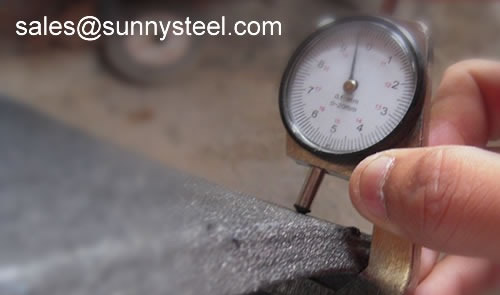We have the professional engineers, measurement the size of steel pipes.

The volume of a pipe equals its surface area times its thickness. Specifically, the surface area of a pipe is the same as a cylinder and equals the number of square inches that can cover the curved sides of the pipe.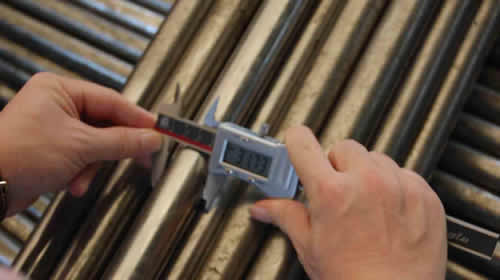Measuring Out Diameter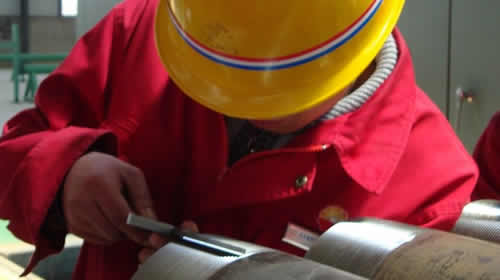Measuring Wall thicknesss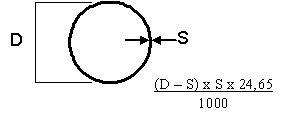T.W.=(D-S)*S*24.65/1000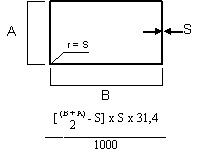T.W.=((B+A)/2-S)*S*31.4)/1000T.W.=12.33*(B+A-2S)*S/1000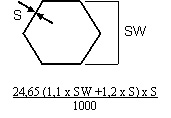T.W.=24.65*(1.1*SW+1.2*S)*S/1000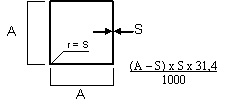T.W.=(A-S)*S*31.4/1000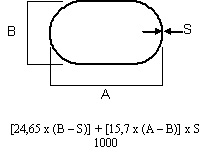T.W.=[(24.65*(B-S))+(15.7*(A-B))*S]/1000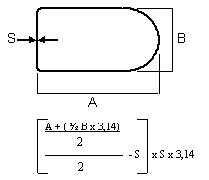T.W.=[(A+(B/2*3.14))/2/2-S]*S*3.14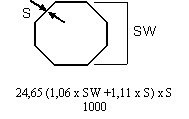T.W.=24.65*(1.06*SW+1.11*S)*S/1000
Gather the necessary information. You will need to know the outside diameter of the pipe. This is the distance of a straight line that runs through the center of the pipe and connects two points on the pipe’s surface. You will also need to know the wall thickness of the pipe. Both of these measurements should be taken in inches. The final thing that you will need to know is the length of the pipe. This last measurement should be taken in feet.

Subtract the pipe’s wall thickness from the pipe’s outside diameter. For example, if you had a pipe with a diameter of six inches and a wall thickness of two inches, you would subtract two from six to get four.